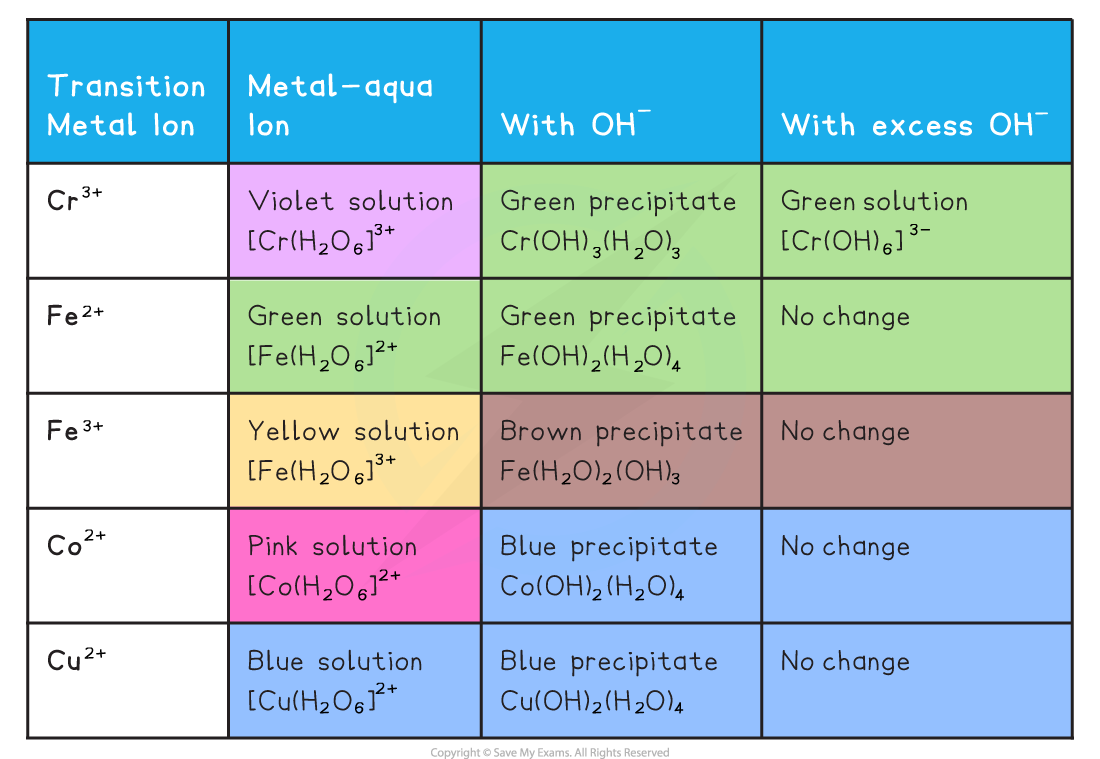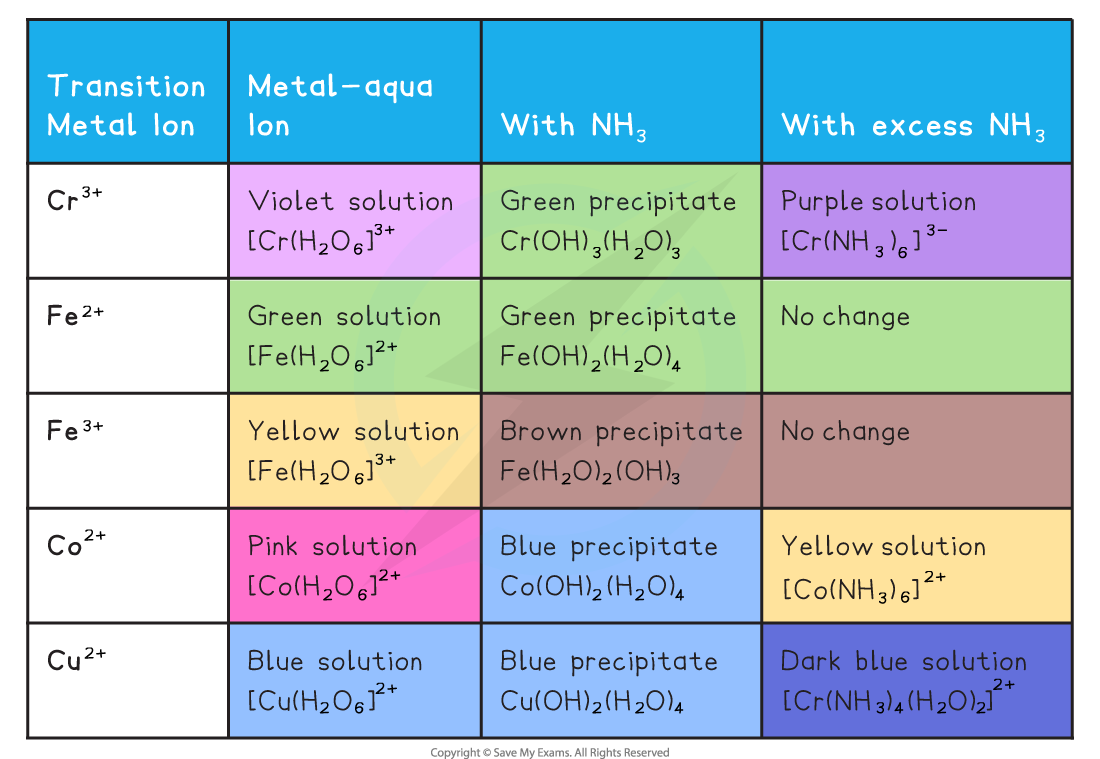# Edexcel A Level Chemistry:复习笔记6.3.3 Reactions of Ions in Aqueous Solution

### Reactions with Hydroxide & Ammonia

• When transition metal ions in aqueous solution react with aqueous sodium hydroxide and aqueous ammonia they form precipitates
• However some of these precipitates will dissolve in an excess of sodium hydroxide or ammonia to form complex ions in solution

The Reactions of Aqueous Transition Metal Ions with Aqueous Sodium Hydroxide• Examples of ionic equations for the reactions in the table above
• [Fe(H2O)6]2+ (aq) + 2OH- (aq) → [Fe(H2O)4(OH)2] (s) + 2H2O (l)
• [Cu(H2O)6]2+ (aq) + 2OH- (aq) → [Cu(H2O)4(OH)2] (s) + 2H2O (l)
• [Fe(H2O)6]3+ (aq) + 3OH- (aq) → [Fe(H2O)3(OH)3] (s) + 3H2O (l)

The Reactions of Aqueous Transition Metal Ions with Ammonia• Examples of ionic equations for the reactions in the table above
• [Fe(H2O)6]2+ (aq) + 2NH3 (aq) → [Fe(H2O)4(OH)2] (s) + 2NH4+ (aq)
• [Cu(H2O)6]2+ (aq) + 2NH3 (aq) → [Cu(H2O)4(OH)2] (s) +2NH4+ (aq)
• [Fe(H2O)6]3+ (aq) + 3NH3 (aq) → [Fe(H2O)3(OH)3] (s) +3NH4+ (aq)
• [Cu(H2O)4(OH)2]2+ (aq) + 4NH3 (aq) → [Cu(H2O)2(OH)4] (aq) + 2H2O (l) + 2OH- (aq)
• Solutions of metal aqua ions react as​ acids ​with aqueous ammonia, whilst some react further with excess ammonia​
• Initially, ammonia acts as a​ base​ to remove one H+ ion per ammonia molecule used
• With excess ammonia, some metal ions undergo ​ ligand substitution ​with NH3

#### Exam Tip

It is easiest to remember the formulas of the precipitates by remembering that the number of OH- ions substituted is the same as the value of the charge on the initial ion

### Ionic Equations

#### Reaction with limited OH- and limited NH3

• The bases OH- and ammonia when in limited amounts form the same hydroxide precipitates.
• They form in deprotonation acid base reactions
• For example, consider the reaction that occurs when aqeuous sodium hydroxide is added to copper(II) sulfate solution

[Cu(H2O)6]2+ (aq) + 2OH- (aq) → [Cu(H2O)4(OH)2] (s) + 2H2O (l)

• This seems like a ligand substitution reaction - two hydroxide ions replacing two water molecules
• However this is actually a deprotonation reaction - two hydroxide ions removing hydrogen ions from two of the water ligands converting them into water molecules
• The two ligands that have lost hydrogen ions are now hydroxide ligand

#### Reaction with excess OH-

• From above, we have seen how hydrated transition metal ions can be deprotonated by adding a base such as aqueous sodium hydroxide to form a metal hydroxide precipitate
• For example

[Cr(H2O)6]3+ (aq) + 3OH- (aq) → [Cr(H2O)3(OH)3] (s) +3H2O (l)

• When an excess of sodium hydroxide is added further deprotonation takes place

[Cr(H2O)3(OH)3] (s) + 3OH- (aq) → [Cr(OH)6]3- (aq) + 3H2O (l)

• In this reaction, chromium(III) hydroxide acts as an acid, as it is reacting with a base
• Chromium(III) hydroxide can also act as a base because it can react with acids as follows

[Cr(H2O)3(OH)3] (s) + 3H+ (aq) → [Cr(H2O)6]3+ (aq)

• A metal hydroxide that can act as both an acid and a base is called an amphoteric hydroxide
• This is an example of amphoteric behaviour

#### Reaction with excess NH3

• With excess NH3 ligand substitution reactions occur with Cu, Co and Cr and their precipitates dissolve
• The ligands NH3 and H2O are similar in size and are uncharged
• Ligand exchange occurs without a change of co-ordination number for Co and Cr
• For example when excess aqueous ammonia is added to a copper(II) hydroxide precipitate it dissolves forming a deep blue solution

[Cu(H2O)4(OH)2] (s) + 4NH3 (aq) → [Cu(NH3)4(H2O)2]2+ (aq) + 2H2O (l) + 2OH- (aq)

• This is a ligand substitution - four ammonia molecules replace two water molecules and two hydroxide ions
• In these reactions NH3 is acting as a Lewis base donating an electron pair